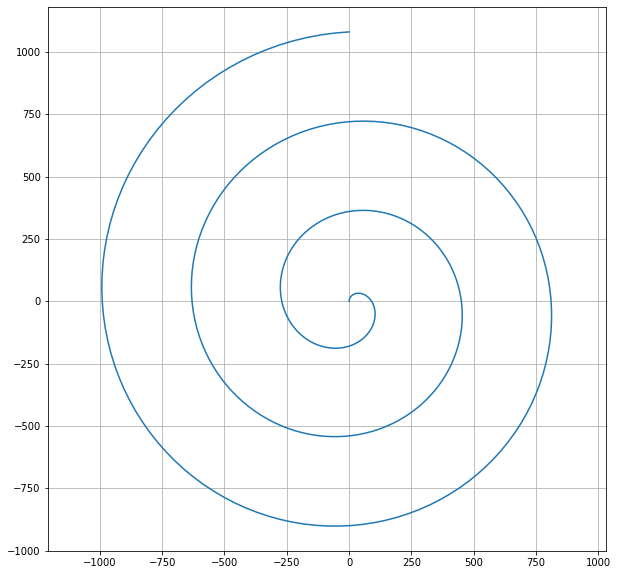# Matplotlib Course For Beginner with Project

## Matplotlib Course For Beginners with Projects

In this course we are going to learn how to plot various graph in matplotlib.pyplot using python

Assumption:
• You are using Jupyter Notebook
• You already have installed all dependencies used in this course (now by default all the dependecies are installed just in case if it not installed type pip install matplotlib in jupyter cell and run [note first try to run the code if its not work then try to install it]).
• You have basic knowledge of python
• Project list below (Mostly 2d and 3d project related to mechanical eginnering )

1. Simple Graph Plotting
import matplotlib.pyplot as plt

x = [1,5,6,7,8]
y = [8,4,5,2,3]
plt.plot(x,y)
[<matplotlib.lines.Line2D at 0x7fde95167290>]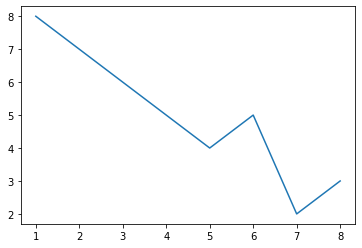2. import matplotlib.pyplot as plt
plt.plot([1,5,7,8,9])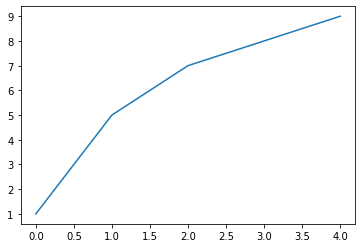3. MultiLine Plots
import matplotlib.pyplot as plt

x = [1,2,3,4]
y = [9,8,7,4]
x1 = [4,5,6,7]
y1 = [9,6,3,4]

plt.plot(x,y,x1,y1)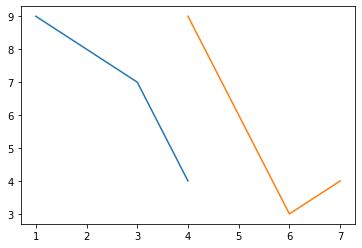4. Grid , Axis and adding Labels
import matplotlib.pyplot as plt

x = [1,5,6,7,8]
y = [8,4,5,2,3]
plt.plot(x,y)
plt.grid(True)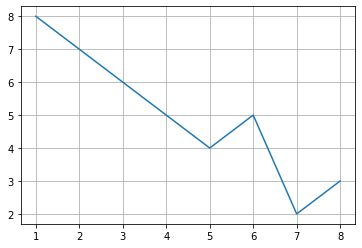import matplotlib.pyplot as plt

x = [1,5,6,7,8]
y = [8,4,5,2,3]
plt.plot(x,y)
plt.grid(True)
plt.axis([-1,10,0,15])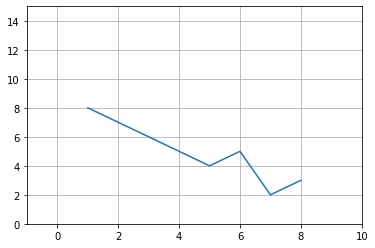import matplotlib.pyplot as plt

x = [1,5,6,7,8]
y = [8,4,5,2,3]
plt.plot(x,y)
plt.xlabel("x-axis")
plt.ylabel("y-axis")
plt.title("Simple Graph")
plt.grid(True)
plt.axis([-1,10,0,15])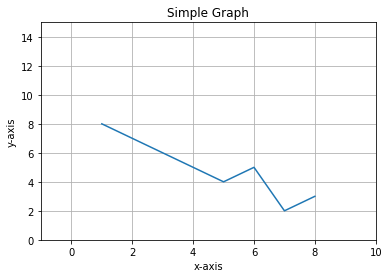5. Legend

import matplotlib.pyplot as plt
import numpy as np
t = np.linspace(1,10,10)
plt.plot(t,t**2,label="square")
plt.plot(t,t,label="linear")
plt.legend()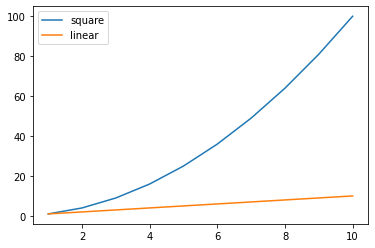6. Styling in Graph

import matplotlib.pyplot as plt
import numpy as np
t = np.linspace(1,10,10)
plt.plot(t,t**2,'#E7429B',label="square")
plt.plot(t,t,'b',label="linear")
plt.legend()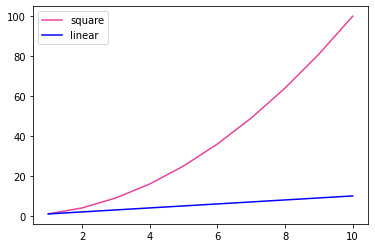import matplotlib.pyplot as plt
import numpy as np
t = np.linspace(1,10,10)
plt.plot(t,t**2,'--',label="square")
plt.plot(t,t,'-.',label="linear")
plt.legend()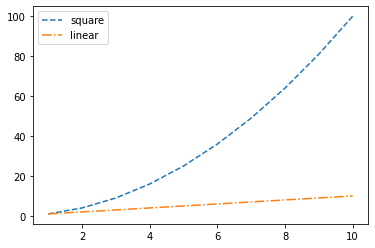7. Scatter Plot

import matplotlib.pyplot as plt
import numpy as np
N = 100
colors = np.random.rand(N)
x = np.random.rand(N)
y = np.random.rand(N)
scale = (10*x*y)
plt.scatter(x,y, s= scale , c = colors)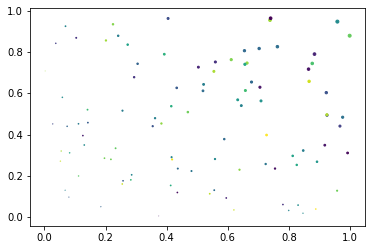8. Pie Chart

import matplotlib.pyplot as plt
x = [20,30,50,60]
y = ["milk","fish","cake","chips"]
plt.pie(x,labels=y)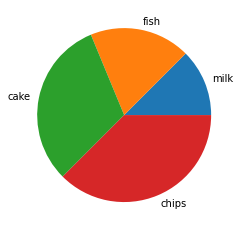import matplotlib.pyplot as plt
x = [20,30,50,60]
y = ["milk","fish","cake","chips"]
explode = [0,0,0.3,0]
plt.pie(x,labels=y ,explode = explode)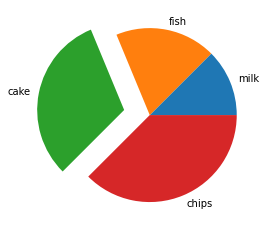# Simple Test base  on Matplotlibsimple Sin Graph plottingimport matplotlib.pyplot as pltimport numpy as npplt.figure(figsize=(10,10))t = np.linspace(0,360,360)x = np.sin(np.radians(t))plt.grid('on')plt.plot(t,x)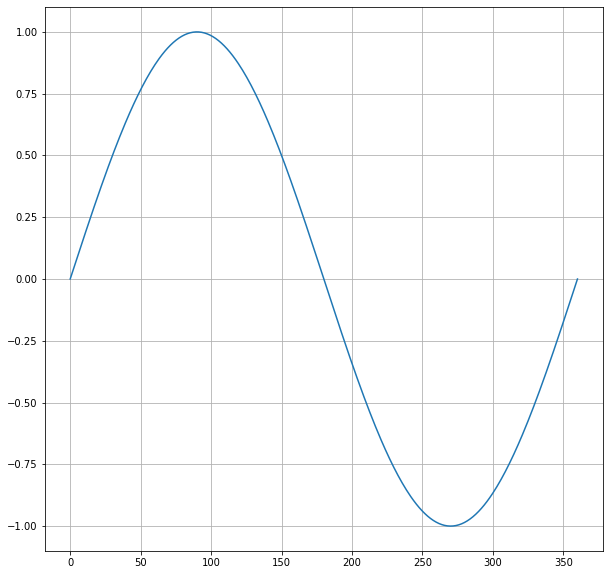Plot a Circle in Python

import matplotlib.pyplot as plt
import numpy as np
plt.figure(figsize=(10,10))
t = np.linspace(0,360,360)  # theta
plt.xlabel('x-axis')
plt.ylabel('y-axis')
plt.title('Circle ')
plt.grid('on')
plt.plot(x,y)
plt.axis('equal')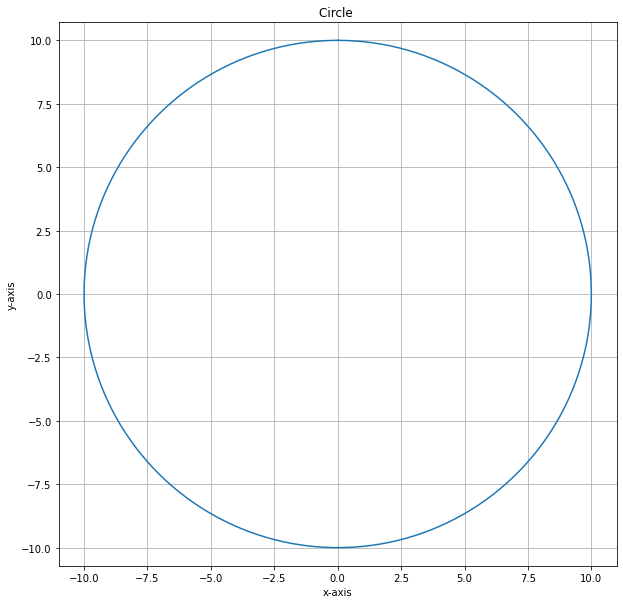Simple Spiral Graph Plotting in Python matplotlib.pyplot

import matplotlib.pyplot as plt
import numpy as np
plt.figure(figsize=(10,10))
t = np.linspace(0,1080,360)  # theta
plt.axis('equal')